# C. Details of the Model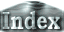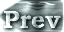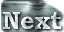### e. Moist Convective Adjustment Scheme

The treatment of moist convection in ordinary atmospheric GCMs involves an approximation that the water vapor content of the atmosphere is small. However, when the value of solar constant increases, the water vapor content in the atmosphere increases significantly. Therefore, it is not appropriate to use this approximation in this case. In the present study, we adopt a convective adjustment scheme that does not include the approximation that the atmospheric water vapor content is small. The details of this convective adjustment scheme will be described below.

The adiabatic condition for air parcels consisting of water vapor and dry air can be expressed as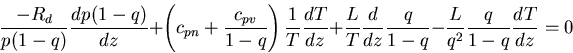If this equation is discretized for the case of cpn=cpv, which is the case under consideration in the present study, the discretized form of the adiabatic equation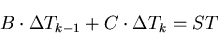is obtained. Here,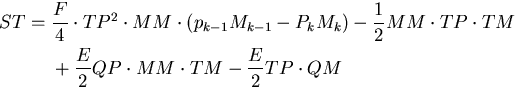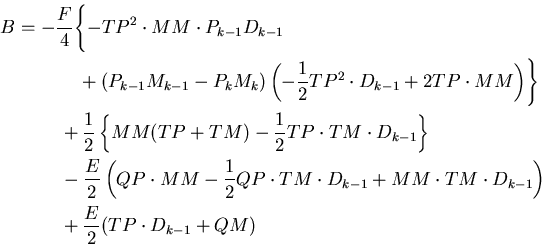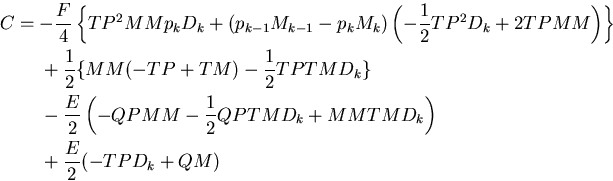where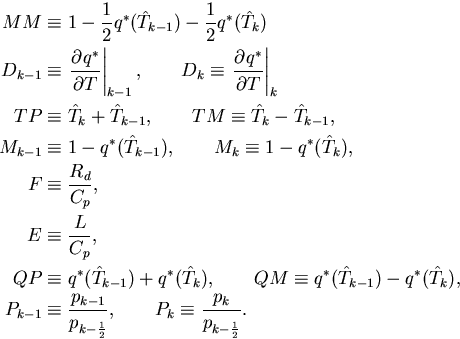Using the discretized adiabatic equation above, the temperature structure in the 1D model is determined and the moist convection adjustment scheme is constructed.

#### Method for Constructing an Adiabat in the One-Dimensional Model

To obtain an adiabatic structure in the 1D model, Tk and qk = q*(Tk) are determined starting from the bottom with Tg and q*(Tg) up to the upper-most layer in such a way as to fulfill the adiabatic equation. In practice, with given Tk-1 and qk-1 , an arbitrary initial estimate value is assigned to Tk and the deviation from the actual Tk is evaluated from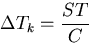in order to determine Tk. Then, q*k is determined from the saturated vapor pressure curve.

In the 3D calculations, the temperature and water vapor profiles are adjusted to the profiles that are determined by the moist adiabat at points meeting the following conditions:

1. 1.unstably stratified
2. 2.saturated or supersaturated

In the course of the adjustment, in addition to the adiabatic conditions, conditions for the conservation of moist static energy between the pre- and post-adjustments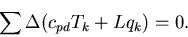will be used. Discretization of this equation leads to a discretized form of the conservation equation for moist static energy: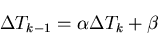Here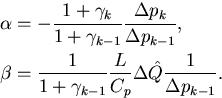where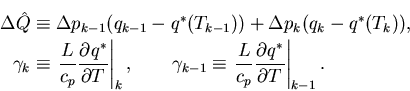By substituting the discretized conservation equation for moist static energy into the discretized adiabatic equation, the following equation can be obtained: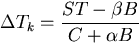From this equation and the discretized conservation equation for moist static energy, Δ Tk-1 and Δ Tk are obtained, which are then used to determine the specific humidity as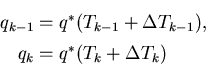The adjustments above are made in each layer in order starting at the lowest layer and repeated up to ten times.

 C.e. Moist Convective Adjustment Scheme